LET'S DO YET ANOTHER STAGGERED CIRCUIT, THIS TIME BETWEEN EACH 4TH STATION.

So, we'll now start from station 1A again, then miss two stations and fix onto the 4th station along the path.

1A TO 3A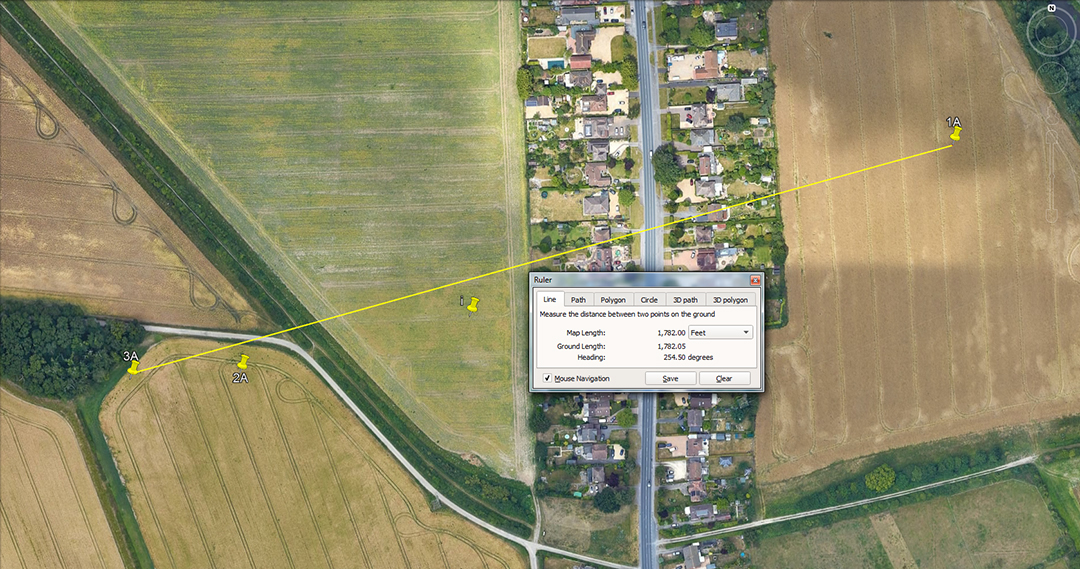The distance is 1782-feet and the return angle is 74.25-degrees.

A mathematical progression based upon 1782 goes:

1782, 3564, 5346, 7128, 8910, 10692, 12474, 14256, 16038, 17820 ... 28512 ... 35640 ... etc.

These numbers are based upon increments of either 2.475-inches, 24.75-inches, 2.475-feet (29.7-inches), or 24.75-feet (297-inches) and in 1782-feet there would be: 8640 X 2.475-inches; 720 X 2.475-feet; 864 X 24.75-inches; 72 X 24.75-feet.

With the full equatorial circumference of the Earth configured to be 22000 miles of 5940-feet (130680000-feet), this also equaled 24750-miles of 5280 or 7920 leagues of 16500-feet each.

3A TO 6A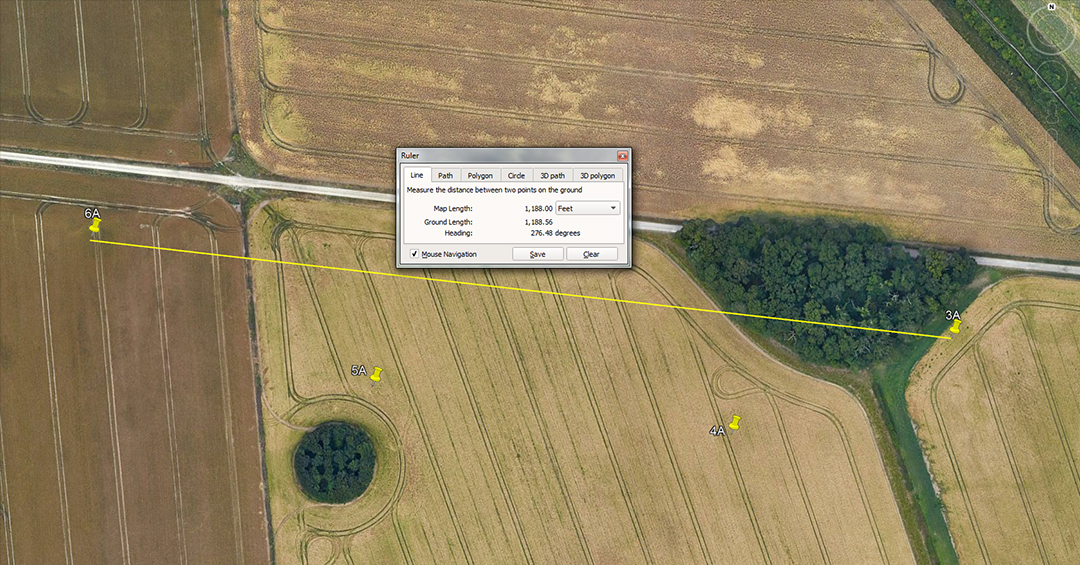The distance is 1188-feet and the angle is 276.48-degrees.

A mathematical progression based upon 1188 goes:

1188, 2376, 3564, 4752, 5940, 7128, 8316, 9504, 10692, 11880 ... 13068 ... 17820 ... 19008 ... 28512 ... 29700 ... 59400 ... 130680000.

Again we have divisions of the navigational system based upon the Scottish mile of 5940, all the way up to the equatorial circumference of the Earth in feet, which can be read as 22000 scottish miles or 24750 English miles.

A mathematical progression based upon 276.48 goes:

276.48, 552.96, 829.44, 1105.92, 1382.4, 1658.88, 1935.36, 2211.84, 2488.32 ... 4147.2 ... 6912 ... 12441.6 ... 20736 ... 24883.2.

Again, we have divisions of the 24883.2-mile equatorial circumference of the Earth. However, if this means the "true" size of the Earth of 24883.2 X 5280-miles the circumference reading is 131383296-feet, of which 276.48-miles is 1/90th in miles of 5280-feet or 1/80th in miles of 5940-feet.

6A TO 9A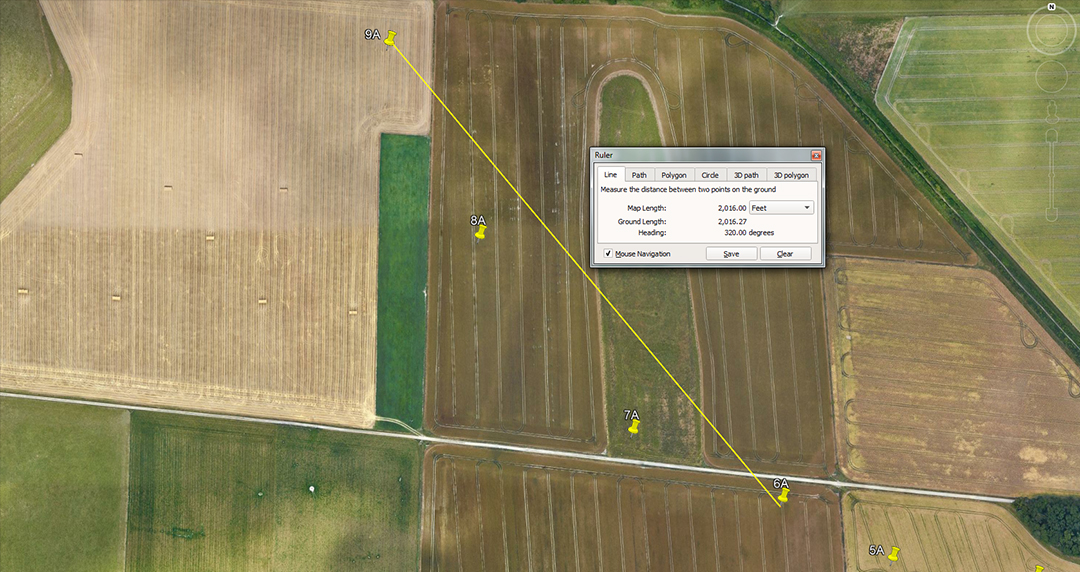The distance is 2016-feet and the angle is 320-degrees. The reverse angle is 140-degrees.

The distance of 2016-feet is 1/3rd of 1-minute of equatorial arc under the 24883.2-mile (of 5250-feet) reading.

A mathematical progression based upon 14 goes:

14, 28, 42, 56, 70, 84, 98, 112, 126, 140, 154, 168 ... 210 ... 224 ... 252 ... 280 ... 336 ... 350 ... 364 ... 378 ... 756 ... 2016 ... 3024 ... 6048, etc.

This string was very important for week, month, year calculations under the Sabbatical Calendar, which was primarily based upon 42 (divisible by both 6&7). The agrarian calendar could run for 7 solar years @ 13-months of 28-days (364-days), accruing 1.25 days of error each year until the end of the Sabbatical Year, where 9-days (8.75) of intercalary days were added to correct the calendar count.

The above progression also provides Great Pyramid values, as well as 6048, which (in feet) equals 1-minute of equatorial arc.

9A TO IV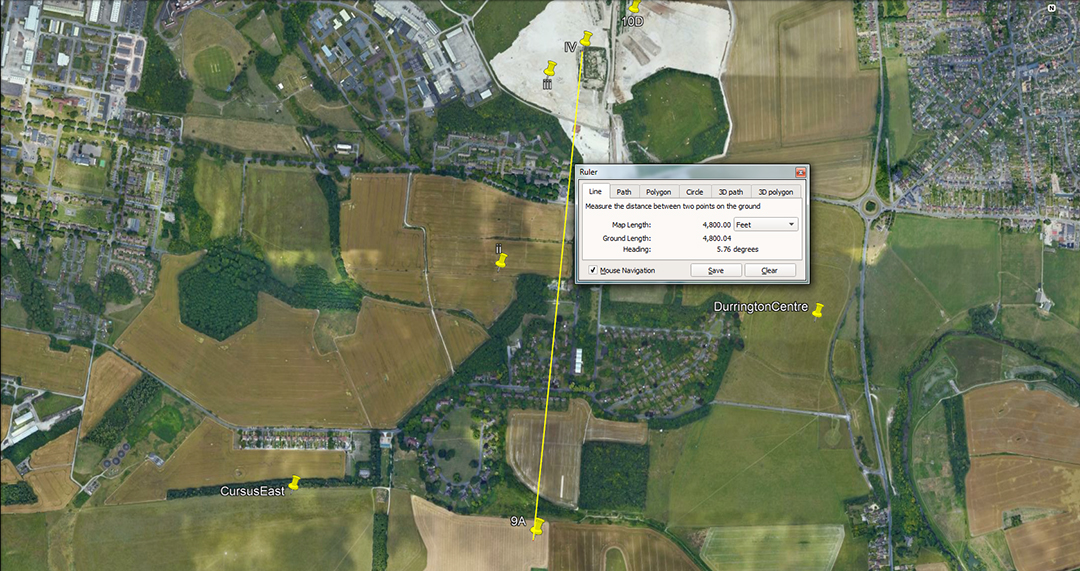The distance is 4800-feet and the angle is 5.76-degrees.

A mathematical progression based upon 48 goes,

48, 96, 144, 192, 240, 288, 336, 384, 432, 480, 528, 576, 624, 672, 720, 768 ... 864 ... 1008 ... 1056 ... 1152 ... 1200 ... 1296 ... 1728 ... 2016 ... 3024 ... 6048 ... 25920, etc.

These are strong navigational numbers or relate to periods within the Precession of the Equinoxes.

The degree angle sets up the following progression:

576, 1152, 1728, 2304, 2880, 3456, 4032, 4608, 5184, 5760, 6336, 6912 ... 8640 ... 9216 ... 10368 ... 13824 ... 15552 ... 19008 ... 20160 ... 20736 ... 25920 ... 31104 ... 36288 ... 124416 ... 248832.

This is a dynamic string, power-packed with useful numbers to do with the equatorial circumference of the Earth, Precession of the Equinoxes, etc.

IV TO 12D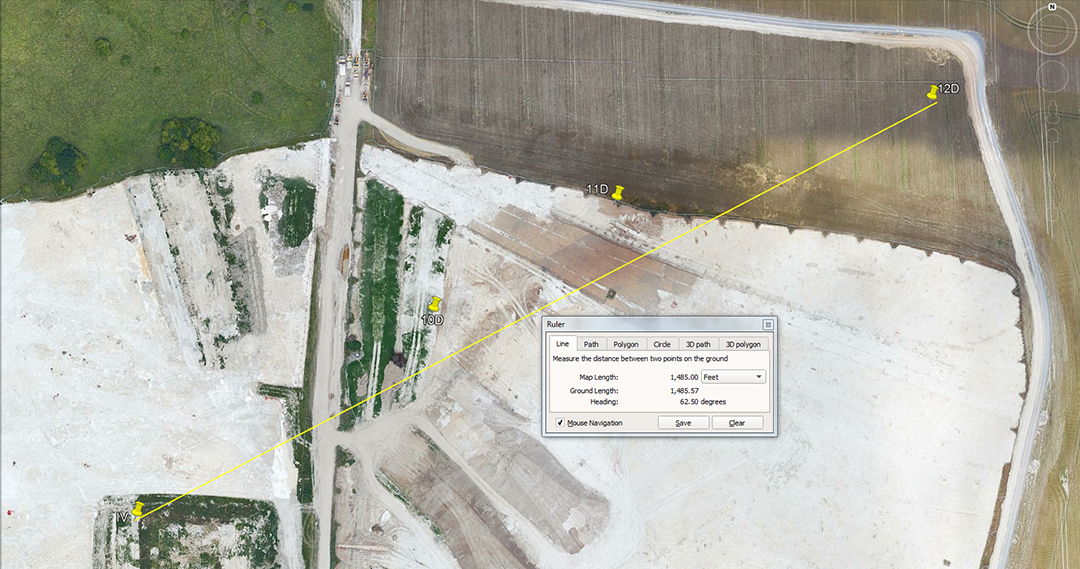The distance is 1485-feet and the angle is 62.5-degrees.

The distance of 1485-feet relates to the Scottish mile of 5940-feet:

1485, 2970, 4455, 5940, 7425, 8910, 10395, 11880, 13365, 14850 ... 17820 ... 29700 ... 35640 ... 59400 ... 65340 ... 130680000

There would be 22000 X 5940-feet long Scottish miles in 130680000-feet or 24750 English miles of 5280-feet.

A mathematical progression based upon 62.5 goes:

625, 1250, 1875, 2500, 3125, 3750, 4375, 5000, 5625, 6250, 6875, 7500, 8125, 8750, 9375, 10000 ... 11250 ... 12500 ... 16875 ... 20625 ... 22500 ... 26250, etc.

This is a somewhat more obscure string, but one that produced many useful numbers for many calculation applications. In the lineup whole number decimal values are being reduced by 1/16th parts.

12D TO 14D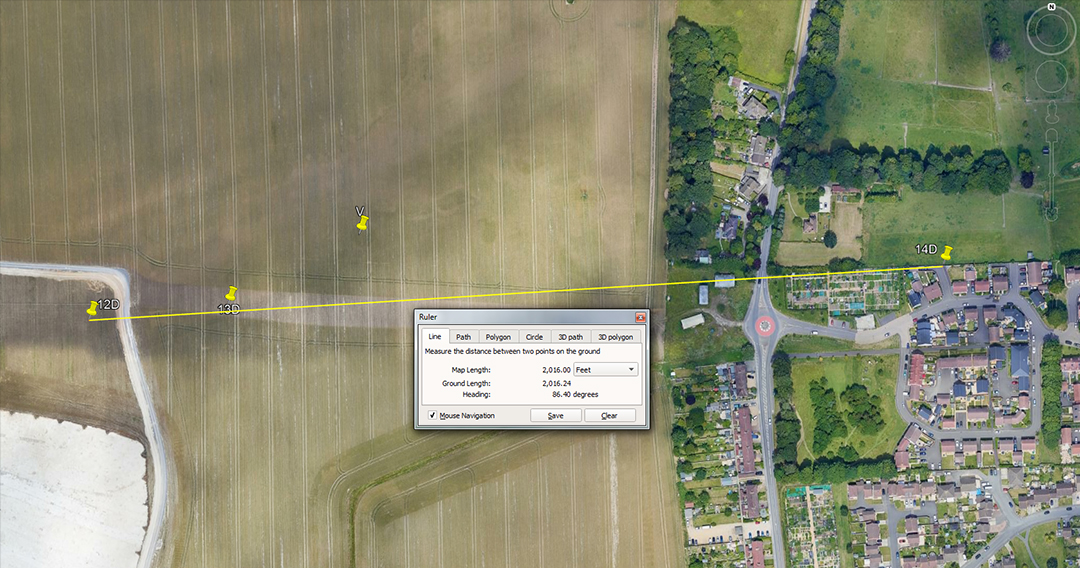The distance is 2016-feet and the angle is 86.4-degrees.

The distance is, of course, 1/3rd of 1-minute of equatorial arc (6804-feet) under the system that used the mile of 5250-feet. This distance would also have been read as 2016.66666-feet or 1/3rd of 6050-feet, the second navigational system encoded into the Great Pyramid, which used a mile of 5280-feet.

The angle code progression is found in the relationship between 13D & 14 D.

SO, LETS GO AROUND AGAIN USING THE SAME STAGGERED METHOD, BUT THIS TIME STARTING FROM THE 2ND STATION ON THE PATH.

i TO 4A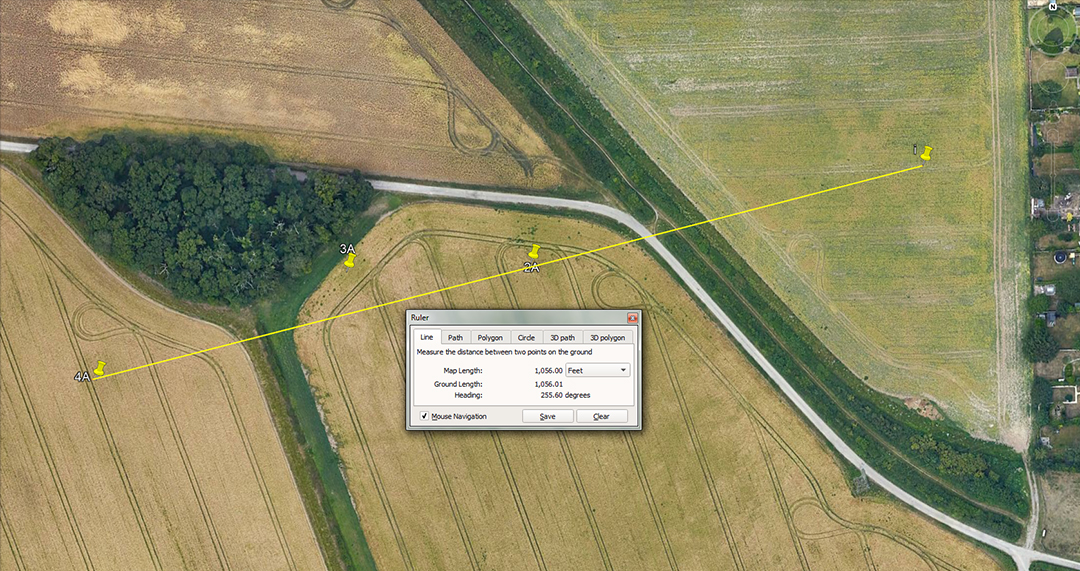The distance is 1056-feet and the angle is 75.6-degrees return. The distance value would also have been read as 1050-feet or 10-reeds.

The sum of 1056-feet is 1/5th of a mile of 5280-feet or there would be 5.625 increments of 1056-feet in a Scottish mile of 5940-feet.

The angle code relates to the 756-feet length of the Great Pyramid, with one side length equal to 1/8th of 1-minute of equatorial arc.

The mathematical progression for this goes:

756, 1512, 2268, 3024, 3780, 4536, 5292, 6048, 6804, 7560 ... 9072 ... 11340 ... 13608 ... 18900 ... 27216 ... 36288 ... 362880, etc.

Highlighted are 1/8th, 1/2, 1-minute of arc and 1-degree of equatorial arc for the navigational method that used the mile of 5250-feet..

The same angle would have coded 75.625, setting up a progression of:

756.25, 1512.5, 2268.75, 3025, 3781.25, 4537.5, 5293.75, 6050 ... 6806.25 ... 7562.5 ... 9075 ... 11343.75 ... 18906.25 ... 27225 ... 36300 ... 363000.

Again, highlighted are 1/8th, 1/2, 1-minute of arc and 1-degree of equatorial arc for the navigational method that used the mile of 5280-feet.

4A TO 7A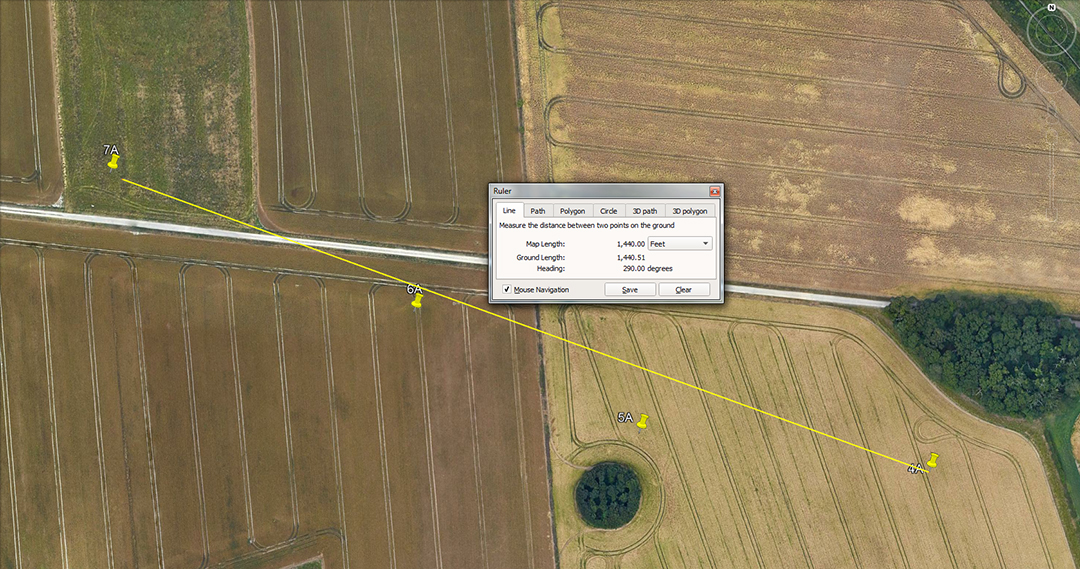The distance code appears to be 1440-feet to the southeastern side of the 7A shaft and 1485-feet to the northwestern side. The return angle is 110-degrees.

A mathematical progression based upon 144 goes:

144, 288, 432, 576, 720, 864, 1008, 1152, 1296, 1400, 1584, 1728 ... 2016 ... 2160 ... 2304 ... 2592 ... 2880 ... 3024 ... 3456 ... 3600 ... 4032 ... 5184 ... 6048 ... 20736 ... etc.

Highlighted are the values that relate to 1-second of equatorial arc (100.8-feet), 1/3rd of 1-minute of equatorial arc (2016), 2/3rds and 1-minute of arc (4032) and 1-minute of arc (6048). The value 20736 is in mnemonic reference to the 20.376-inch Egyptian Royal cubit (1.728-feet).

The 1485 code is covered in the relationship between V to 12D.

7A TO CURSUS EAST POND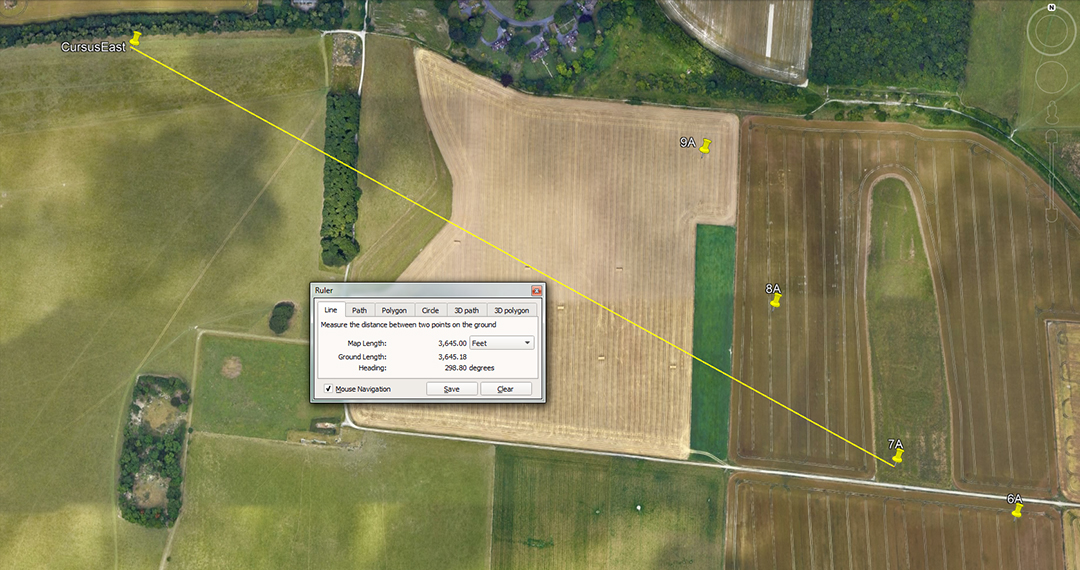The distance is 3645-feet and the return angle is 118.8-degrees.

A mathematical progression based upon 3645 goes:

3645, 7290, 10935, 14580, 18225, 21870, 25515, 29160 ... 34560 ... 58320 ... 72900 ... 116640 ... 131220 ... 466560 ... 131220000.

Under the Roman system of navigation, their foot was, as stated, 11.664-inches and 5000 such feet was their mile (4860-feet of 12-inches). It was known in mediaeval times that 75 Roman miles was 1-degree of equatorial arc (364500-feet of 12-nches or 375000 Roman feet of 11.664-inches) meaning a full circumference was configured mathematically to be 131220000-feet of 12-inches or 135000000 Roman feet.

In the above lineup, 7290 feet of 12-inches would equal 7500 Roman feet; 58320-feet of 12 inches would equal 60000 Roman feet or 12000 Roman paces.

For overland marching it seems plausible that the Romans would have used the more easily calculated foot of 11.66666-inches and, if so, their value for the equatorial circumference would come to 131250000-feet of 12-inches or 135000000 Roman feet or 25000 Greek miles of 5250-feet of 12-inches.

Of course, all of these increments were encoded into the Durrington Walls landscape up to 2000-2500-years before there were any hints or emerging vestiges of Greek or Roman civilisations.

7A TO ii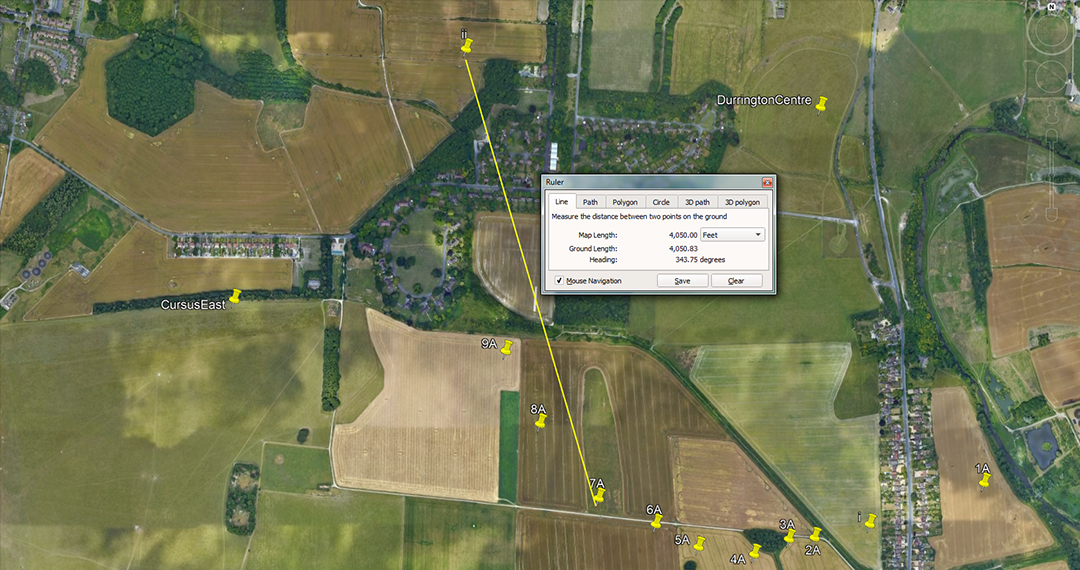The distance is 4050-feet and the angle is 343.75-degrees.

A mathematical progression based upon 405 goes:

405, 810, 1215, 1620, 2025, 2430, 2835, 3240, 3645, 4050 ... 4860 ... 5670 ... 6480 ... 7290 ... 8100 ... 8505 ... 17010 ... 34020 ... 68040 ... 25515, etc.

This is essentially a lunar string. In the 2551.5 days (7.2 lunar years) tracked within the lunisolar Sabbatical Calendar, there would be 63 X 40.5-days (972-hours). Alternatively, there would be 168 X 40.5-days in the 6804-day lunar nutation cycle.

The angle code is 343.75-degrees and a mathematical progression based on this goes:

343.75, 687.5, 1031.25, 1375, 1718.75, 2062.5, 2406.25, 2750 ... 3437.5 ... 4125 ... 5156.25 ... 5500 ... 6187.5 ... 6875 ... 7562.5 ... 11000 ... 16500 ... 24750.

This string shows divisions within the 16500-feet English league (3.125-miles of 5280-feet). There would be 7920-leagues in 24750-miles. In a league of travel the sum of 343.75-feet would be 1/48th. It would also equate to 200 Egyptian Royal cubits of 20.625-inches. Also, with the earth configured to be 24750-miles in circumference, 1-degree of arc would be 68.75-miles.

ii TO 10D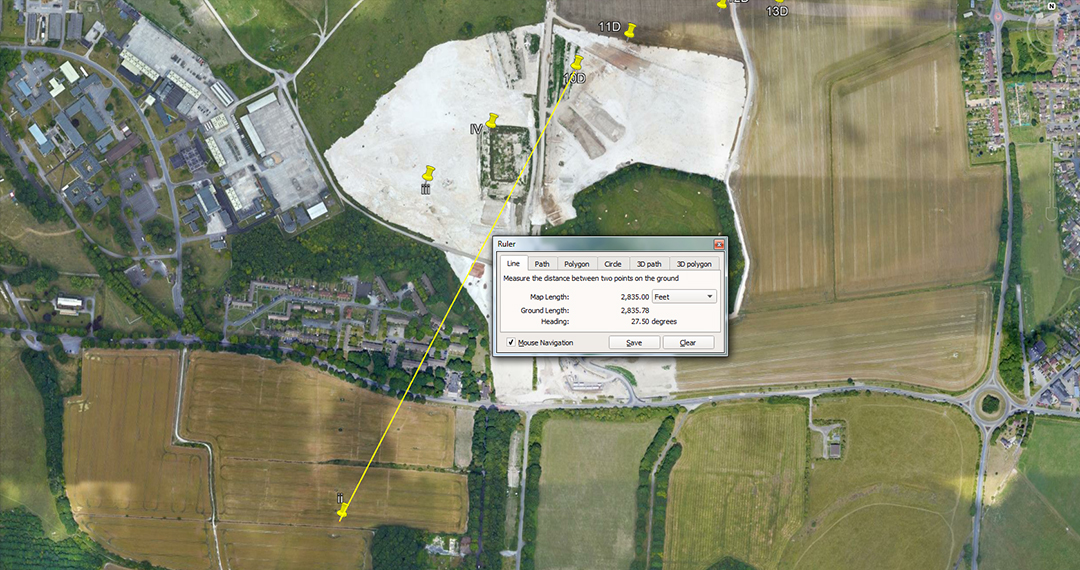The distance is 2835-feet and the angle is 27.5-degrees.

The distance is the same as doing 1 circumnavigation of the Khafre Pyramid of Egypt (2835-feet) or if considered to be 2835-days, that would equate to 8-lunar years.

The degree angle sets up the following progression:

27.5, 55, 82.5, 110, 137.5, 165, 192.5, 220, 247.5, 275, 302.5, 330, 357.5 ... 440 ... 495 ... 550 ... 605 ... 660 ... 687.5 ... 880 ... 907.5 ... 990 ... 2062.5 ... 2475 ... 24750.

In this string we have divisions of the furlong or furrowlong of which 27.5-feet would be 1/24th. Alternatively, 27.5-feet would be 1/192nd of a mile of 5280-feet, 1/216th of a Scottish mile of 5940-feet or 1/600th of a league of 16500-feet.

10D TO 13D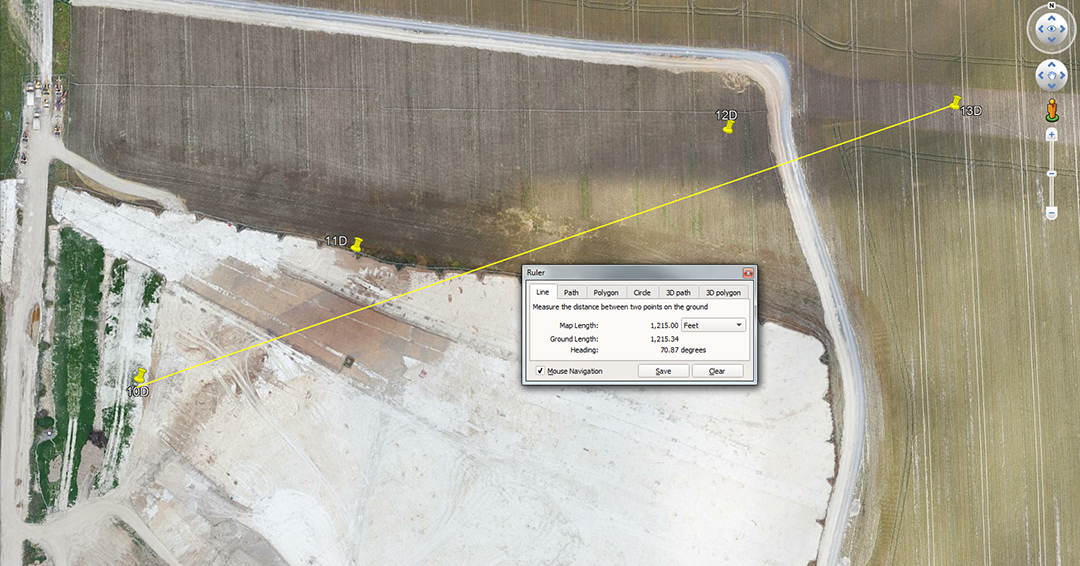The distance is 1215-feet and the angle is 70.875-degrees. The tutorial would also have included distances of 1200 and 1209.6-feet respectively, as well as the angle of 72-degrees (252-degrees return).

A mathematical progression about the lunar codes for 1215 is adequately covered in the shaft distance between 7A to ii.

The degree angle is, once again, in homage to the Khafre Pyramid of Egypt's base length of 708.75-feet, coding 2-lunar years of 354.375-days.

13D TO 15D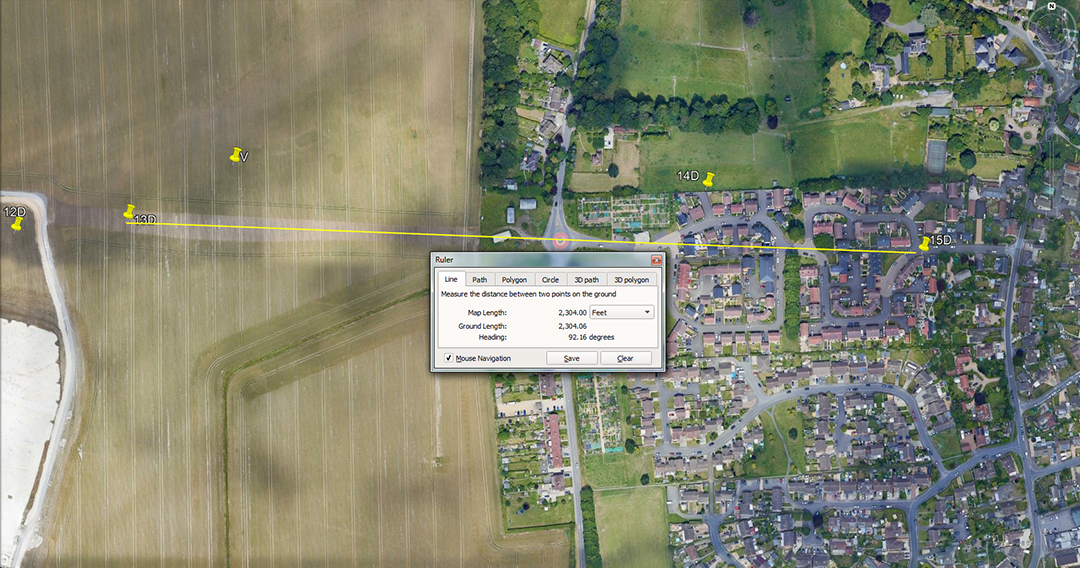The distance is 2304-feet and the angle is 92.16-degrees (return angle 272.16-degrees).

A mathematical progression based upon 2304 is covered in the distance between shafts ii to IV.

The codes for 92.16 are covered in V to 14D.

The sum of 27216 feet would be 1/48th of the 130636800-feet equatorial reading.

13D TO DURRINGTON CENTRE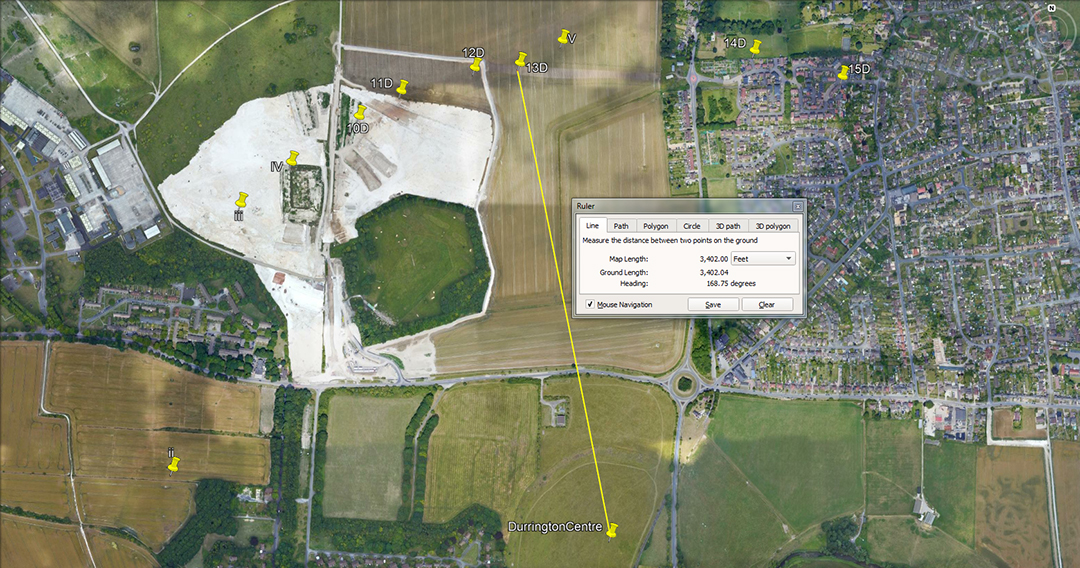The distance is 3402-feet and the angle is 168.75-degrees.

A mathematical progression based upon 340.2 goes:

340.2, 680.4, 1020.6, 1360.8, 1701 ... 3402 ... 6804, etc., is simply in homage to the 6804-day lunar nutation cycle. In this cycle the moon moves to its most extreme positions up and down the horizon, in the same vein as an annual summer or winter solstice of the sun, except in the case of the moon the elapsed time is 18.613-years.

The lunar coding related to 168.75 is covered in the relationship between shafts 8A to ii.

Continue